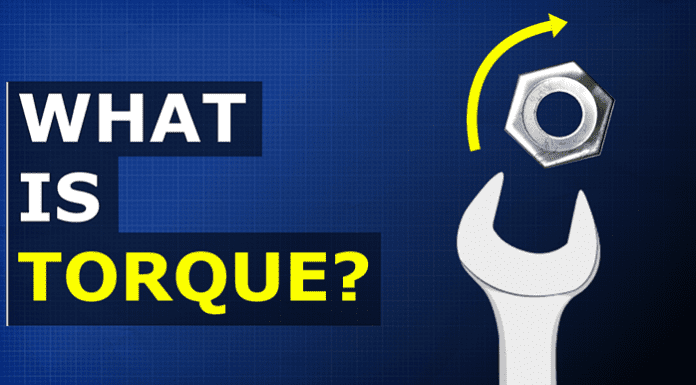# What is Torque?

Learn the basics of Torque. In this article we learn what is torque, how it is used with worked examples and gears to illustrate the principle.

1Learn the basics of Torque. In this article we learn what is torque, how it is used with worked examples and gears to illustrate the principle.

Torque is the measurement of the force which causes something to rotate around a point.  Think of a wrench and a nut that’s seized up. Using a small wrench it’s very hard to free the nut. Using a long wrench will make it much easier. That’s because of torque. If we use a 30cm wrench, and applied 90 newtons of force, we have 0.3 metres x 90 newtons equals 27 newton meters of torque. However, if we applied the same 90 newtons of force to a wrench that was 60 centimetres long then we would get 0.6 metres x 90N = 54N.m. So from this simple formula, we can see we have more force acting on the nut by using a longer wrench. Essentially we are using a larger circle to turn a smaller circle. By changing the size, we change the speed and the torque. A low gear provides low speed but high torque, a high gear gives high speed by low torque.

## Why Do We Need Gears?

If you have ever ridden a bike, you will know it is very difficult to start peddling in a high gear. So we need to start in a low gear to get the bike moving. At a certain point our legs are spinning very fast, but we cant go any faster. So we need to change to another higher gear. Once we reach a steep hill, we need to move to a lower gear. The same with a car, we start in our lowest gear number, and work our way up as the vehicle increases in speed. Then, we change down as we drive up a hill.

#### 1 COMMENT

1.hi

Nice, but you left out pure torque.